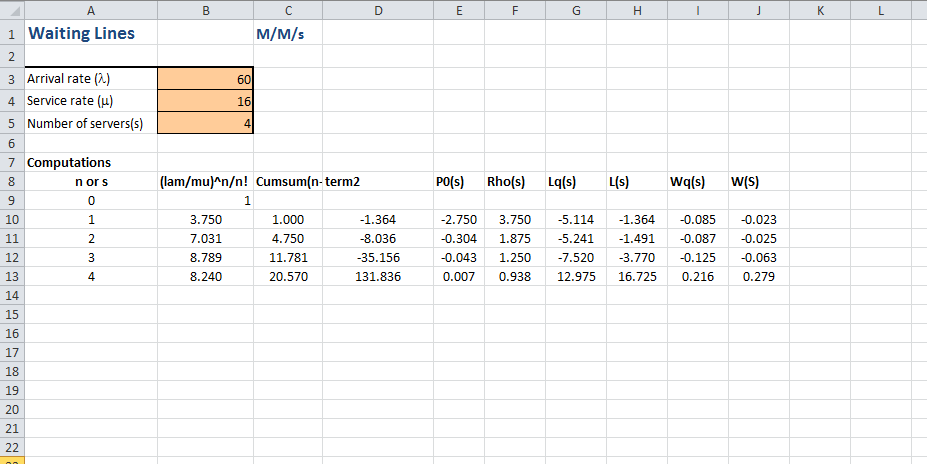# Question & Answer: Question #1 has been answered on this website, but when i go to do the same process…..

This is question #1:

1) During busy times, 60 potential customers per hour arrive at the booth (assume a Poisson distribution). A booth worker takes 3.75 minutes, on average, to meet the information needs of a potential customer (assume an exponential distribution).

Don't use plagiarized sources. Get Your Custom Essay on
Question & Answer: Question #1 has been answered on this website, but when i go to do the same process…..
GET AN ESSAY WRITTEN FOR YOU FROM AS LOW AS \$13/PAGE

This is question #2:

2) During slack times, 10 potential customers per hour arrive at the booth (assume a Poisson distribution). A booth worker takes 3.75 minutes, on average, to meet the information needs of a potential customer (assume an exponential distribution).

1) Arrival rate, λ = 60 customers per hour

Service rate, μ = (1/3.75)*60 = 16 customers per hour

Arrival rate is more than the service rate, therefore multiple booth workers are required.

Minimum number of booth workers required = 60/16 = 3.75 ~ 4 workers (rounded-up to integer, because worker cannot be in fraction)

This is M/M/s queue model with s=4

With 4 workers, Average waiting time in line, Wq = 0.22 hour = 13 minutes (approx)

Calculation of waiting time is following2) Arrival rate, λ = 10 customers per hour

Service rate, μ = (1/3.75)*60 = 16 customers per hour

Arrival rate is less than the service rate, therefore 1 worker is enough.

This is M/M/1

Average waiting time in line, Wq = λ/(μ*(μ-λ)) = 10/(16*(16-10)) = 0.1042 hour = 6.25 minutes (approx)